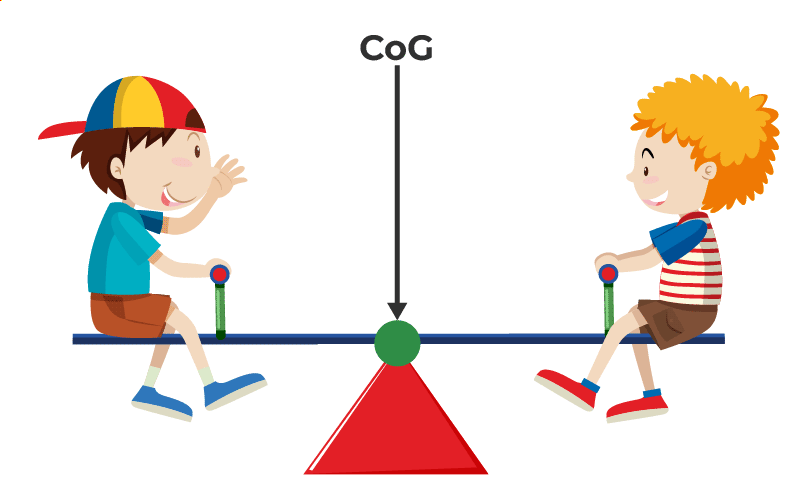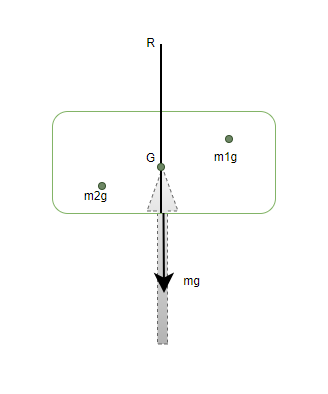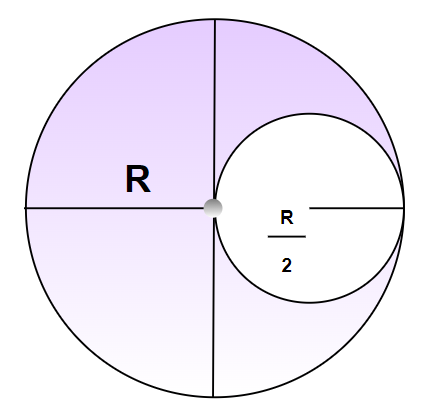Open In App
Related Articles
• CBSE Class 11 Physics Notes

# Centre of Gravity

Centre of Gravity is one of the fundamental concepts in the study of gravitational force. Engineers and Scientists while dealing with mechanics and gravity often come across solid bodies which can’t be represented by point masses such as celestial objects. In those cases, it is assumed as well as proved that there is a certain point where all the mass and gravity of the body is concentrated and also acts on a certain. From this use case, the concept of the Centre of Mass and Centre of Gravity emerges.In this article, we will learn about the concept of the Centre of Gravity with topics including its definition, formulas and the difference between the centre of gravity and the centre of mass. So, let’s start learning about the concept of the Centre of Gravity.

## Centre of Gravity Definition

Theoretically, the centre of gravity is considered to be a point where the weight of the body acts. Finding this point is very essential in bodies that have large sizes and often non-uniform mass distribution. This point allows us to predict the motion of such bodies under the influence of gravity. If a body is balanced by this point, it is rotational and translational equilibrium. Consider the example of the body given in the figure below,The figure shows cardboard being balanced by a pencil kept below it. The tip of the pencil applies a reaction force on the point which keeps the cardboard in translational equilibrium. It is also in rotational equilibrium, it can be verified by the fact that the object does not have any angular acceleration.

## Formula for Centre of Gravity

Let us assume that the cardboard is made up of very small individual point masses m1, m2, m3… Gravity is acting on all these point masses creating torque around the point. The Centre of Gravity (CoG) is located such that the total torque on CoG due to forces on individual point masses is zero. If ri is the position vector of the ith particle with respect to the CoG, then the torque on it due to the gravitational force is given by,For a point that is the centre of gravity, the total torque about that point must be zero,Notice that in the equation above, the gravitational force “g” is the same for every point. It can come out of the equation as a common factor to all the terms.Since g is a constant and non-zero. It can be concluded that,### Difference between the Centre of Gravity and Centre of Mass

It is assumed that there is no difference between the Centre of Gravity and the Centre of Mass, but that’s not the case. There are some differences between both concepts which are listed as follows:

## Solved Examples on Centre of Gravity

Example 1: Two point masses, m1 = 5Kg and m2 = 2Kg, are located at x = 2 m and x = 6 m respectively. Find the Centre of gravity.

Solution:

Formula for the Centre of gravity is equal to centre of mass when gravity is constant

xcm =m1 = 5Kg,  m2 = 2Kg and x = 2 m and x = 6 m.

M = m1 + m2

⇒ M = 5 + 2 = 7

xcm =⇒ xcm =⇒ xcm =⇒ xcm =Example 2: Twopoint masses, m1 = 5Kg and m2 = 2Kg, are located at y = 10m and y = -5 m respectively. Find the Centre of gravity.

Solution:

Formula for the Centre of gravity is given by,

ycm =m1 = 5Kg,  m2 = 2Kg and y = 10m and y = -5 m.

M = m1 + m2

⇒ M = 5 + 2 = 7

ycm =⇒ ycm =⇒ ycm =⇒ ycm =Example 3: Twopoint masses, m1 = 1Kg and m2 = 2Kg, are located at vector a = 6i + 4j and vector b = -5i + 2j respectively. Find the Centre of gravity.

Solution:

Formula for the Centre of gravity in the vector notation is given by,

rcm =m1 = 1Kg,  m2 = 2Kg and a = 6i + 4j, b = -5i + 2j

M = m1 + m2

⇒ M = 1 + 2 = 3

rcm =⇒ rcm =⇒ rcm =⇒ rcm =⇒ rcm =Example 4: Twopoint masses, m1 = 4Kg and m2 = 2Kg, are located at vector a = i + j and vector b = -i + j respectively. Find the Centre of gravity.

Solution:

Formula for the Centre of gravity in the vector notation is given by,m1 = 4Kg,  m2 = 2Kg and a = i + j, b = -i + j

M = m1 + m2

⇒ M = 4 + 2 = 6Example 5: A disk of radius R/2 is removed from a bigger disk of mass M of radius R. Find the Centre of gravity.Solution:

Since the density of the disk is uniform, the weight is uniformly distributed over all the area.

Mass “m” of the removed disk =Let the distance of the centre of mass of the remaining portion be “x”.Example 6: A disk of radius R/4 is removed from a bigger disk of mass M of radius R, in the same manner as the previous figure. Find the Centre of gravity.

Solution:

Since the density of the disk is uniform, the weight is uniformly distributed over all the area.

Mass “m” of the removed disk =Let the distance of the centre of gravity of the remaining portion be “x”.## FAQs on Centre of Gravity

### Q1: What is Centre of Gravity?

The centre of gravity is the point at which the total weight of an object is considered to be concentrated. It is the point where the gravitational force can be assumed to act on the object.

### Q2: Why is Centre of Gravity Important?

The centre of gravity is important because it is the point where the weight of an object is balanced, and any force applied to the object will affect its stability and balance. Understanding the centre of gravity is crucial for designing stable structures, vehicles, and machines.

### Q3: How is Centre of Gravity Determined?

The centre of gravity can be determined experimentally by suspending an object from various points and finding the point where it hangs in perfect balance. It can also be calculated mathematically by finding the weighted average of the positions of all the parts of an object.

### Q4: What Factors Affect Centre of Gravity?

The centre of gravity is affected by the shape, size, and weight distribution of an object. Objects with a larger mass concentrated near one end will have a centre of gravity closer to that end.